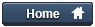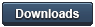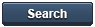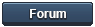### Gammon Forum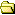Entire forum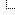MUSHclient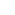Lua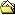PCRE versus RE(LPEG) versus LPEG

PCRE versus RE(LPEG) versus LPEG

It is now over 60 days since the last post. This thread is closed.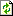Refresh page

Posted by WillFa   USA  (525 posts)bio
Date Tue 24 Feb 2009 09:26 PM (UTC)

Amended on Wed 25 Feb 2009 12:32 AM (UTC) by Nick Gammon

Message
 With the release of MUSHClient 4.40, the lua scripters have access to a new tool: LPEG. How do they compare to one another? Nick gave us a sample of what LPEG and the RE.lua can do, but I was curious about a more practical benchmark. Let's test it against some more practical data. On the mud I play, 3 Kingdoms, my HP bar looks like: `````` HP: 1346/1351 K: 497/537 SP: 289/289 V: 91% [[ AG:379 O:362 ab RF PE:238 PH:139 B HS:50 ]] P: 8 T:98% | 811372/2914265 (45%) `````` Some of the considerations I've needed to make in my trigger were: Not all pieces are always there: the "P: #" and the "T:98%" are only present if I'm performing a song, or in combat. Everything between the "[[" and "]]" are abilities. They are in no fixed order, nor will the entire section be there if no abilities are up. Since PCRE does not handle recursion well, nor repeated captures, a secondary pass to actually parse the abilites is needed. **NB: The benchmark in this test was done thru the rex library in the Lua script engine. It is not indicative of the MushClient C++ implementation of the trigger engine. ``` pcre = rex.new [=[^ HP\: (?P\d+)\/(?P\d+) K\: (?P\d+)\/(?P\d+) SP\: (?P\d+)\/(?P\d+) V\: (?P\d+)\% (?:[[ (?.*?) ]] )?(?PP\: (?P(?:\d+|\*)))? (?PT\:((?P\d+)\%?|\w+))? \| (?P\d+)\/(?P\d+) \((?P.+)\%\)\$]=] ``` `````` function ParseHPFlags(wilds) vars = {mVoice = 100} vars.ActivePowers={} for k,v in pairs(wilds) do vars[k] = tonumber(v) or v end percent(vars, "HP") percent(vars, "SP") percent(vars, "Karma") vars.pVoice = vars.cVoice if vars.Powers then for Flag,Duration in vars.Powers:gmatch( "([^ :]+):?(%d*)") do vars.ActivePowers[Flag] = tonumber(Duration) or true end end end function percent(self, val) self["p" .. val] = math.floor((self["c"..val]/self["m"..val])*100) end `````` The resulting table looks like: `````` tprint(vars) --> 1=1346 2=1351 3=497 4=537 5=289 6=289 7=91 8="AG:379 O:362 ab RF PE:238 PH:139 B HS:50" 9="P: 8" 10=8 11="T:98%" 12="98%" 13=98 14=811372 15=2914265 16=45 "ActivePowers": "AG"=379 "B"=true "HS"=50 "O"=362 "PE"=238 "PH"=139 "RF"=true "ab"=true "BadGuyHealth"=98 "GXPtoNextLevel"=2914265 "GXPtoNextPercent"=45 "GXPtoSpend"=811372 "Performing"="P: 8" "Powers"="AG:379 O:362 ab RF PE:238 PH:139 B HS:50" "cHP"=1346 "cKarma"=497 "cSP"=289 "cVoice"=91 "duration"=8 "inCombat"="T:98%" "mHP"=1351 "mKarma"=537 "mSP"=289 "mVoice"=100 "pHP"=99 "pKarma"=92 "pSP"=100 "pVoice"=91 `````` Okay. It works. It's not pretty. The resulting table is mostly flat. Running pcre:match(hp) and the supporting function 100,000 times on my laptop took 12 seconds.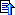top

Posted by WillFa   USA  (525 posts)bio
Date Reply #1 on Tue 24 Feb 2009 09:27 PM (UTC)

Amended on Tue 24 Feb 2009 10:36 PM (UTC) by WillFa

Message
 RE(LPEG) My main concerns with the PCRE pattern is that I don't have a lot of control over how the data is returned, and the length and complexity of that pattern was difficult (a 330 character line is not easy to edit). Looking again at the HP bar, there are several logical sections. It's much easier to work with just those sections than the entirety of the line. The RE syntax is a bit easier to work with, since you can just deal with these pieces and then build them up to make the whole. I'll explain the syntax a bit more in a reply. `````` RElpeg = re.compile ([==[ HPBar <- ( {:Stats::}{:Prots::}?{:Song::}?{:Combat::}?{:GXP::} ) ->{} stats <- ( ' HP: ' {:cHP: :} '/' {:mHP: :} ' K: ' {:cKarma: :} '/' {:mKarma: :} ' SP: ' {:cSP: :} '/' {:cSP: :} ' V: ' {:cVoice: :} '%' )->{} prots <- ' [[ ' {::}+->{} ' ]]' prot <- ( ({[%a*]+} ':' ) / ({'RA'} ) / {[%a*]+} ) ->SplitKeys/ ' ' performing <- ( ' P: ' {:Duration: :}/ ' ' ) ->{} combat <- (' T:' {:EnemyHealth: :} '%' / ' T:' {:EnemyHealth: %a+ :} / ' ') ->{} gxp <- (' | ' {:G2spend: :} '/' {:GXPtoNextLevel: :} ' (' {:GXPtoNextPercent: :} '%)' ) ->{} num <- {%d+}->tonumber ]==] , { SplitKeys = function (k,v) return {[k] = tonumber(v) or true } end , tonumber = tonumber, }) `````` This may look completely foreign at first glance, but there are similarities to PCRE to help get you adjusted. With PCRE, where you would use (?P x ) for a named capture, in RE you use {:name: x :}. In PCRE, (capture) is {capture} in RE. In PCRE, (?: ) is a non-capturing group, in RE it's simply ( ) In PCRE, | is the or character. In RE, it's / In PCRE, every non-special character is a literal. In RE, literals are always quoted. So in PCRE (?:this|that) would be ('this' / 'that') In RE, < > denote a named sub-pattern. They're defined in the format name <- pattern In RE, ->{} means put the captures into an empty table. In RE, ->func means put the captures into the parameters of a call to func (spiffy!) so what does all that get us? `````` foo = RElpeg:match(hp) tprint (foo) --> "Combat": "EnemyHealth"=98 "GXP": "G2spend"=811372 "GXPtoNextLevel"=2914265 "GXPtoNextPercent"=45 "Prots": 1: "AG"=379 2: "O"=362 3: "ab"=true 4: "RF"=true 5: "PE"=238 6: "PH"=139 7: "B"=true 8: "HS"=50 "Song": "Duration"=8 "Stats": "cHP"=1346 "cKarma"=497 "cSP"=289 "cVoice"=91 "mHP"=1351 "mKarma"=537 `````` Hey, that's looking a bit more robust. I didn't like the layout of 'foo.Prots.AG = 379, foo.Prots.O = 362' . I just wanted them all in the same table named Prots. Also, the percent values are no longer in the table, like they were when added in the loop that followed the PCRE parsing. Hmm, not the right tool for what I want then. How did it perform? 100000 calls in 8 seconds.top

Posted by WillFa   USA  (525 posts)bio
Date Reply #2 on Tue 24 Feb 2009 09:27 PM (UTC)

Amended on Wed 25 Feb 2009 04:54 PM (UTC) by WillFa

Message
 LPEG LPEG syntax takes a little bit to get used to. It's all math operators that are used in a way that makes sense when you get the hang of it, but will trip you up at first. LPEG doesn't use .. for concatenation ("hi" .. " " .. "there" in plain Lua, 'hi ' 'there' in RE). It uses * I finally started remembering that when I realized that repetitions in LPEG (i.e. PCRE {1,2}) are exponents ^ So a * a is like a^2 . That's just math. Okay. Since LPEG uses math operators, "Please Excuse My Dear Aunt Sally" applies when parsing. (ie. order of operations, priority, etc) ( ) are still grouping. ^n are repetitions, tho ^n is the same as PCRE's {n,} (at least n matches.) ^-n are like PCRE's {,n} (at most n matches) * is concatentation. / is apply pattern to a function call. + is OR. wth? Well, somethings gutta be or, and with the multiplication -> exponent analogy, it grows on ya. p-q is a logical disjunction. (everything in p except q) -p is negation (not p, or everything except p) So a * a + b is "a twice or b" not "a and either a or b" use parens if you need to clarify, as usual. The documentation explains the functions in more detail, so I'll gloss over most of them. In my code below I use the following functions: P for literal text, except the initial compile. V for specifying a subpattern. (V"name" is in RE) Cg is capture group. Cg(a * a + b, 'foo') is {:foo: a a / b:} in RE. (i.e. to pass more than one argument to a function, or name a table key) Ct is Capture to a table. {a} -> {} in RE S is a set, like [abcde] in PCRE. R is a range, like [a-z] in PCRE. C is a simple capture. (don't need a group, one's fine.) The 2 new concepts below are Cc is a Constant capture. It matches the empty string but has the captured value specified. and Cf Folding Capture. Recurse down a function with all matches. `````` do lpeg.locale(lpeg) local P, V, Cg, Ct, Cc, S, R, C, Cf, Cb = lpeg.P, lpeg.V, lpeg.Cg, lpeg.Ct, lpeg.Cc, lpeg.S, lpeg.R, lpeg.C, lpeg.Cf, lpeg.Cb local digit = lpeg.digit local alpha = lpeg.alpha local flag = lpeg.alpha + lpeg.S"*" local function Tn (s) return lpeg.Cg(lpeg.V(s), s) end local function namekeys ( t, k, v) t[k] = tonumber(v) or v return t end local function num (name) return Cg(digit^1 / tonumber, name) end local function perc (x) x.percent = math.floor((x.current/x.max)*100) return x end local function group (name) return Cg(Ct( num"current" * P'/' * num"max")/ perc, name) end local function vper (x) x.percent = x.current return x end LPEG = P { "top", top = Ct( Tn"Stats" * Tn"Prots" * Tn"Performing" * Tn"Combat" * Tn"GXP" ) , Stats = Ct(V"HPtoken" * V"Ktoken" * V"SPtoken" * V"Vtoken"), HPtoken = P" HP: " * group"HP" * P" ", Ktoken = P" K: " * group"Karma" , SPtoken = P" SP: " * group"SP" , Vtoken = Cg(Ct( P" V: " * num"current" * P "%" * Cg(Cc(100), "max") )/ vper, "Voice") , Prots = P" [[ " * Cf(Ct("") * V"abil"^1 , namekeys) * P" ]]", abil = V"dur" + V"present" + P" " * V"abil", dur = Cg( C(alpha^1) * P":" * C(digit^1) ), present = Cg( C(flag^1) * Cc(true)), Performing = Ct( (P" P: " * num"Duration") + P" "), Combat = Ct( ( P" T:" * V"ehealth" * P" " ) + P" " ), ehealth = ( num"enemyHealth" * P"%" ) + Cg(alpha^1, "EnemyHealth"), GXP = Ct( P"| " * num"toSpend" * P"/" * num"toNext" * P" (" * num"nextPercent" * P"%)" ), } end `````` Okay! I think that actually reads a bit more easily without worrying about <- and ->, sure you still have ambiguously named variables, but the general coding structure is cleaner. So, new things in here over RE (and almost all of RE should be new. Sorry for not explaining them better.) I've used some functions to work as macros for common "phrases" in my grammar. The Cf function takes an Empty table and the two captures returned from (either or ) and calls namekeys with them. it returns that table and then the Cf function uses that returned table instead of the empty table to make a second call to namekeys with more results from subsequent matches to (thus a space and more or ) So what do we end up with? `````` Me = LPEG:match(hp) tprint(Me) --> "Combat": "enemyHealth"=98 "GXP": "nextPercent"=45 "toNext"=2914265 "toSpend"=811372 "Performing": "Duration"=8 "Prots": "AG"=379 "B"=true "HS"=50 "O"=362 "PE"=238 "PH"=139 "RF"=true "ab"=true "Stats": "HP": "current"=1346 "max"=1351 "percent"=99 "Karma": "current"=497 "max"=537 "percent"=92 "SP": "current"=289 "max"=289 "percent"=100 "Voice": "current"=91 "max"=100 "percent"=91 `````` Exactly what I want. A Robust, heirarchy instead of a flat table. How does this complexity perform? 100,000 calls in 8 seconds again. Still faster than PCRE in this application and giving me exactly what I want.top

Posted by Worstje   Netherlands  (899 posts)bio
Date Reply #3 on Tue 24 Feb 2009 10:23 PM (UTC)
Message
 You're giving me a headache. Of course, it being past midnight doesn't help me any. :) First of all, I hate your indenting. There is structure to it in some parts, but not in others. I also sincerely dislike the func"blah" notation.. anything used in an expression should use parentheses imo. But that's all superficial. Second, I can't make heads or tails of your example. I don't know your original input (unless it is in there somewhere and I'm reading over it) so I can't really figure out what I am working with either... or wait, I just found it. (Yay for the new reply screen showing stuff in reverse order and my scrolling all the way down by habit.) For now it still remains a mystery to me though. Third, won't this throw out any resemblence of ordering? It seems unimportant in your example, both the resulting table and your matching pattern table thingy do not seem to touch on it. I can imagine quite a few prompts with things on it behaving like a stack of sorts - order of attacking enemies, list of upcoming boosts or whatever. Probably stupid questions that I could answer myself if I took a shot at it when I wake up. :Dtop

Posted by WillFa   USA  (525 posts)bio
Date Reply #4 on Tue 24 Feb 2009 10:41 PM (UTC)

Amended on Tue 24 Feb 2009 10:43 PM (UTC) by WillFa

Message
 Sorry about the indenting. I didn't convert tabs to spaces before copying. It's fixed now. The original input is the 5th line of the first post. It's the first [code] block. It is also followed by why the patterns are the way they are. I specifically wanted keyed tables, and not indexed results. The default behavior is to return ordered lists, as seen in the RE example. Sorry about your headache. Get some sleep, it'll make sense in the morning. (the forum seems to be eating paragraphs at the moment... sorry for the list)top

Posted by Nick Gammon   Australia  (22,928 posts)bio   Forum Administrator
Date Reply #5 on Wed 25 Feb 2009 12:40 AM (UTC)
Message
 Very interesting. I am sure I benchmarked LPEG a while ago but maybe didn't report the results. It was fast enough to not discourage me, and your tests seem to show that it is indeed faster than PCRE. Of course in your example, you could use a simple trigger (like: HP: *) to capture the prompt, and then pass it all to your lpeg parser to break it down in more detail. I am glad you found a use for stuff like Cc, I think I'll have to refer to your examples to improve my knowledge of lpeg. :) Lpeg 0.9 added named capture groups, I don't think that was in 0.8 when I first tested it. I amended your first post to change from a [code] tag to [mono] - that is sometimes more useful for very long lines. At least for the first line, where indenting is not important. - Nick Gammon www.gammon.com.au, www.mushclient.comtop

Posted by WillFa   USA  (525 posts)bio
Date Reply #6 on Wed 25 Feb 2009 02:09 AM (UTC)

Amended on Wed 25 Feb 2009 06:13 AM (UTC) by WillFa

Message
 I'm glad you're intrigued, Nick. The big thing with my example is that the abilities section guarantees that PCRE needs to backtrack, even if you use a non-greedy capture for ?P (everything between the doubled brackets.) I forgot to mention why I used Cc, and found another instance where I should have used it, but forgot about that scenario. In the RE example, the funtion tonum(v) returns tonumber(v) or true. In the LPEG example, the namekeys function returns tonumber() or v. Because of the Cc, I'm sure I'm passing in a non-nil value for v, this allows for bypassing pesky error checking and if I were to reuse the function again, other values won't get trampled. Simple example. The Scenario I forgot, there's a few songs that have an infinite duration. I can hum all day on the mud if I wanted. The mud sends " P: *" for these infinite songs. `````` Performing = Ct( (P" P: " * num"Duration") + Cg(P" P: *" * Cc(1000), "Duration") + P" ") `````` That would match and consume upto the asterisk, and still give me a numerical capture (1000) to test against for other Abilites' duration checks.top

Posted by WillFa   USA  (525 posts)bio
Date Reply #7 on Wed 25 Feb 2009 08:56 AM (UTC)

Amended on Wed 25 Feb 2009 04:56 PM (UTC) by WillFa

Message
 Quote:Third, won't this throw out any resemblence of ordering? It seems unimportant in your example, both the resulting table and your matching pattern table thingy do not seem to touch on it. I can imagine quite a few prompts with things on it behaving like a stack of sorts - order of attacking enemies, list of upcoming boosts or whatever. You have complete control of not only the matching inside an LPEG, but also of the capturing and the returns. Multiple returns: `````` lpeg.locale(lpeg) -- populates Sets for lpeg.digit, lpeg.alpha, etc to the current codepage. patt = (lpeg.C(lpeg.digit^1) * lpeg.space^0)^1 fee, fi, foe, fum = patt:match("2 3 45 68") REpatt = re.compile ([[ multiplereturns <- ({%d+} %s*)+ ]] sweden, denmark, finland, norway = REpatt:match( "1 2 4 5" ) `````` Anonymous table captures: `````` pattT = lpeg.Ct( (lpeg.C(lpeg.digit^1) * lpeg.space^0)^1 ) tprint( pattT:match( "2 4 6 8") ) --> 1 = 2 2 = 4 3 = 6 4 = 8 REpattT = re.compile([[ indexedtable <- ({%d+} %s*)+ -> {} ]]) tprint (REpattT:match( "7 8 9 10" ) --> 1 = 7 2 = 8 3 = 9 4 = 10 `````` Named Captures: The Cg() or {:id: :} syntax only has relevance in a Table capture or when setting up a backreference. `````` pattG = lpeg.Cg( (lpeg.C(lpeg.digit^1) * lpeg.space^0), "Foo") print ( pattG:match("1 2 5 7") -> 8 --the pattern stopped matching at the 8th position, the end of string. pattGOoops = lpeg.Ct( lpeg.Cg( (lpeg.C(lpeg.digit^1) * lpeg.space^0), "Foo") ) tprint ( pattGOoops:match("1 2 5 7") ) -> "Foo" = 1 --(other return values have been truncated.) pattGGood = lpeg.Ct( lpeg.Cg( lpeg.Ct((lpeg.C(lpeg.digit^1) * lpeg.space^0), "Foo") )) tprint ( pattGGood:match("1 2 5 7") ) -> "Foo": 1 = 1 2 = 2 3 = 5 4 = 7 REpattG = re.compile( [[ namedTable <- {:Foo: ({%d}+ %s* )+ ->{} :} ->{} ]] ) tprint ( REpattG:match("1 2 5 7") ) -> "Foo": 1 = 1 2 = 2 3 = 5 4 = 7 ``````top

Posted by Erendir   Germany  (47 posts)bio
Date Reply #8 on Wed 25 Feb 2009 03:42 PM (UTC)

Amended on Wed 25 Feb 2009 04:25 PM (UTC) by Erendir

Message
 to WillFa there are some small mistakes: LPEG section: Quote: ^n are repetitions, tho ^n is the same as PCRE's {,n} (at most n matches.) ^-n are like PCRE's {n,} (at least n matches) vice versa in last post: `````` pattGGood = lpeg.Ct( lpeg.Cg( lpeg.Ct((lpeg.C(lpeg.digit^1) * lpeg.space^0), "Foo") )) tprint ( pattGOoos:match("1 2 5 7") ) `````` should be `````` local pattGGood = lpeg.Ct( lpeg.Cg( lpeg.Ct((lpeg.C(lpeg.digit^1) * lpeg.space^0)^0), "Foo" )) tprint ( pattGGood:match("1 2 5 7") ) `````` Also in RE section, You write Quote: I didn't like the layout of 'foo.Prots.AG = 379, foo.Prots.O = 362' . I just wanted them all in the same table named Prots. But lpeg and lpeg.re are, as documantation says, identically. You just need a bit different grammar :) (I'll try to find it out, tomorrow ;)) upd ok, it was easier, as i thought: in grammar: `````` prots <- ' [[ ' {::}+->FoldListToTable ' ]]' prot <- ( ({[%a*]+} ':' ) / ({'RA'} ) / {[%a*]+} )->SplitKeys/ ' ' `````` functions table: `````` { SplitKeys = function (k,v) return k, tonumber(v) or true end , FoldListToTable = function (...) local t = {} local arg = {...} for i = 1,#arg,2 do t[arg[i]]=arg[i+1] end return t end, tonumber = tonumber, } ``````top

Posted by WillFa   USA  (525 posts)bio
Date Reply #9 on Wed 25 Feb 2009 06:11 PM (UTC)
Message
 Thanks. I fixed the typos in the sample code (I shouldn't try to code at 5am!) and exponent comment. The funny thing is I had the exponents right initially, and then went back and changed them after thinking about it too much.... RE isn't identical to LPEG tho, not all functions have lexigraphical symbols in RE. There's no RE substitution for Cc, nor Cf. `````` prots <- ' [[ ' {::}+->FoldListToTable ' ]]' `````` becomes in LPEG `````` local Cg, V = lpeg.Cg, Lpeg.V Cg(V"prot")^1 / FoldListToTable `````` It's similar to `````` foo = {1,2,3,4,5} function w (...) return {...} end foo = w(0,table.unpack(foo)) --is functionally equivalent to table.insert(foo, 1, 0) `````` Thanks for the help. :)top

Posted by Erendir   Germany  (47 posts)bio
Date Reply #10 on Wed 25 Feb 2009 07:16 PM (UTC)
Message
 Quote:RE isn't identical to LPEG tho, not all functions have lexigraphical symbols in RE. indeed, my wrong. (Don't know why had I thought so. Possibly, in one older version they was identically) Quote:There's no RE substitution for Cc, nor Cf. yes, but it can be easily implemented. Maybe, in 1.0?.. ;)top

Posted by WillFa   USA  (525 posts)bio
Date Reply #11 on Wed 25 Feb 2009 07:50 PM (UTC)
Message
 RE is implememted in Re.lua, which is just an lpeg parser for making other lpegs. If you can decide on a symbol, you can do it yourself. :) {@ @} for Cf or what have you...top

Posted by Erendir   Germany  (47 posts)bio
Date Reply #12 on Wed 25 Feb 2009 08:17 PM (UTC)
Message
 I would rather let Roberto Ierusalimschy do this job -- i have no use for such feature now. I thought about to add ability to name groups with back references (like {:=name: p :} ), but what for, i'm still not using lpeg.re. lpeg is more suitable.top

Posted by WillFa   USA  (525 posts)bio
Date Reply #13 on Wed 25 Feb 2009 08:28 PM (UTC)
Message
 Yea, I thought about that one too which would make the whole FoldListToTable vs Cf thing moot. :)top

Posted by Anaristos   USA  (24 posts)bio
Date Reply #14 on Thu 27 Feb 2014 12:45 PM (UTC)

Amended on Thu 27 Feb 2014 12:46 PM (UTC) by Anaristos

Message
 I found a way to simulate lpeg.Cf(p,f) by keeping the accumulator as an upvalue and calling the accumulating function each time there is a capture: `````` require("re") require("tprint") -- bit = require("bit") -- local bor = bit.bor -- flagman = {} -- function flagman.ctor( ) -- a emulated lpeg.Cf( p, f ) -- local self = {} local flags = 0 -- result buffer. local alist = { Angry = 128 ,Diseased = 64 ,Invisible = 32 ,Hiding = 16 ,Translucent = 8 ,["Golden Aura"] = 4 ,["Red Aura"] = 2 ,["White Aura"] = 1 ,D = 64 ,I = 32 ,H = 16 ,T = 8 ,G = 4 ,R = 2 ,W = 1 } -- local fold = function( f ) -- this function will be called each time there is pattern match. local mask = alist[f] or 0 flags = bor( flags, mask ) return flags end -- local reflags = re.compile ( "flags <- ( '(' {| [^)]+ -> fold |} ')' %s* )*", { fold = fold } ) -- re does not support lpeg.Cf( p, f). However this function can be emulated by making the accumulator an upvalue. function self.getflags( text ) flags = 0 -- initialize result buffer. reflags:match( text ) -- parse input string. return { flags = flags } end -- return self -- end -- setmetatable( flagman, { __call = function( _, ... ) return flagman.ctor( ... ) end } ) `````` a simple use would be: `````` fm = flagman() text = "(Red Aura)(White Aura) A little girl is a little worried about the idea." -- flags = fm.getflags(text) -- -- tprint(flags) will show "flags"= 3 `````` Sic itur ad astratop

The dates and times for posts above are shown in Universal Co-ordinated Time (UTC).

To show them in your local time you can join the forum, and then set the 'time correction' field in your profile to the number of hours difference between your location and UTC time.

73,653 views.

It is now over 60 days since the last post. This thread is closed.Refresh page

Go to topic:           Search the forumtop

Quick links: MUSHclient. MUSHclient help. Forum shortcuts. Posting templates. Lua modules. Lua documentation.

Information and images on this site are licensed under the Creative Commons Attribution 3.0 Australia License unless stated otherwise.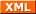Forum RSS feed ( https://gammon.com.au/rss/forum.xml )